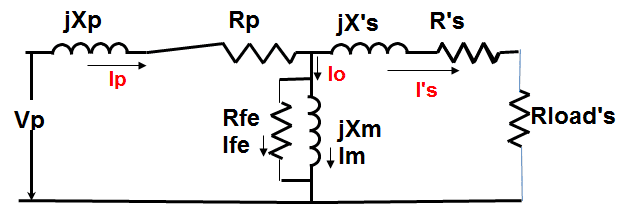# Transformers and resistance

ak33m98
Do transformers change the resistance of the wires inside then when turned on? The reason I ask is because I know that transformers can be used to change the ratios between voltage a current while keeping the power sent in and power sent out roughly equal, but since V=IR this must mean that the resistance in the output/secondary circuit changes as the ratio between V and I does

Doug Huffman
Gold Member
No. Look into reactance and reactive impedance.

meBigGuy
Gold Member
A transformer does not change the power IN/OUT much less do anything based on changing the resistance.

Let's talk perfect 2X step up transformer where there are 2 times the number of turns in the secondary as in the primary. You connect 50V AC to the primary and 50 ohms to the secondary. Since there will be 100V on the secondary (because of the 2:1 stepup) there will be 2A in the load. 2A at 100V = 200W. That means there must be 200W flowing into the primary (where else can the power come from?), or 4A. If you change the resistance to 100 ohms, there will be 1A, 100W so there will only be 100W into the primary of the transformer, or 2A.

So, what ever the power consumed by the secondary load, the power flowing into the primary will be just enough to supply that power. The perfect transformer has no power loss. You could say that the transformer "reflects" it load to the primary based on the turns ratio.

With that in mind, read the wikipedia article.
http://en.wikipedia.org/wiki/TransformerI agree, of course, with meBigGuy.If you look from primary you see Rload modified by ratio (w1/w2)^2
where :
w1=primary winding number of turns
w2=secondary winding number of turns